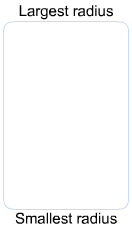# Problem: Rank these ions according to ionic radius.Ca2+     P3-     S2-     Cl-     K+

###### FREE Expert Solution

Total valence electron:

Ca2+ = 20 – 2 = 18

P3- = 15 + 3 = 18

S2- = 16 + 2 = 18

Cl- = 17 + 1 = 18

K+ = 19 – 1 = 18

All of the ions are isoelectric

90% (9 ratings)###### Problem Details

Rank these ions according to ionic radius.Ca2+     P3-     S2-     Cl-     K+